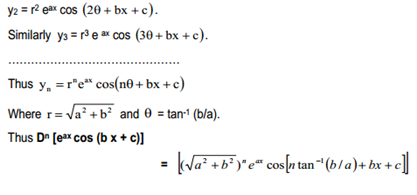Leibnitz Theorem:

We know that the product of two n times differentiable functions is n times differentiable as well. The Leibnitz formula gives an explicit expression for the n-th derivative of the product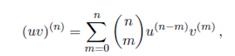Where as usual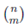is the binomial coefficients " n choose m".

Proof : We use induction with respect to n. For n = 1 the formula is correct. Suppose it is correct for the n-th derivatives and check its correctness for the n 1-st derivatives: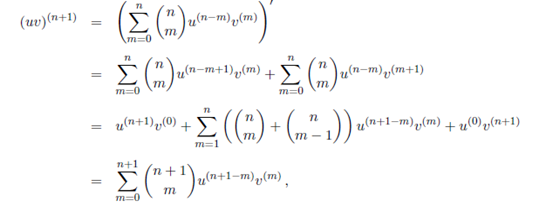Compliting the arguments.

Find the nth derivatives of eax cos(bx c)

Solutions: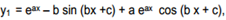by product rule.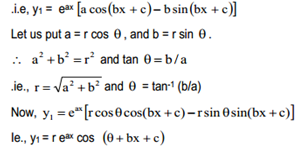Where we have used the formula Cos A Cos B - Sin A Sin B = Cos ( A B ). Differentiate again and simplifying as before.